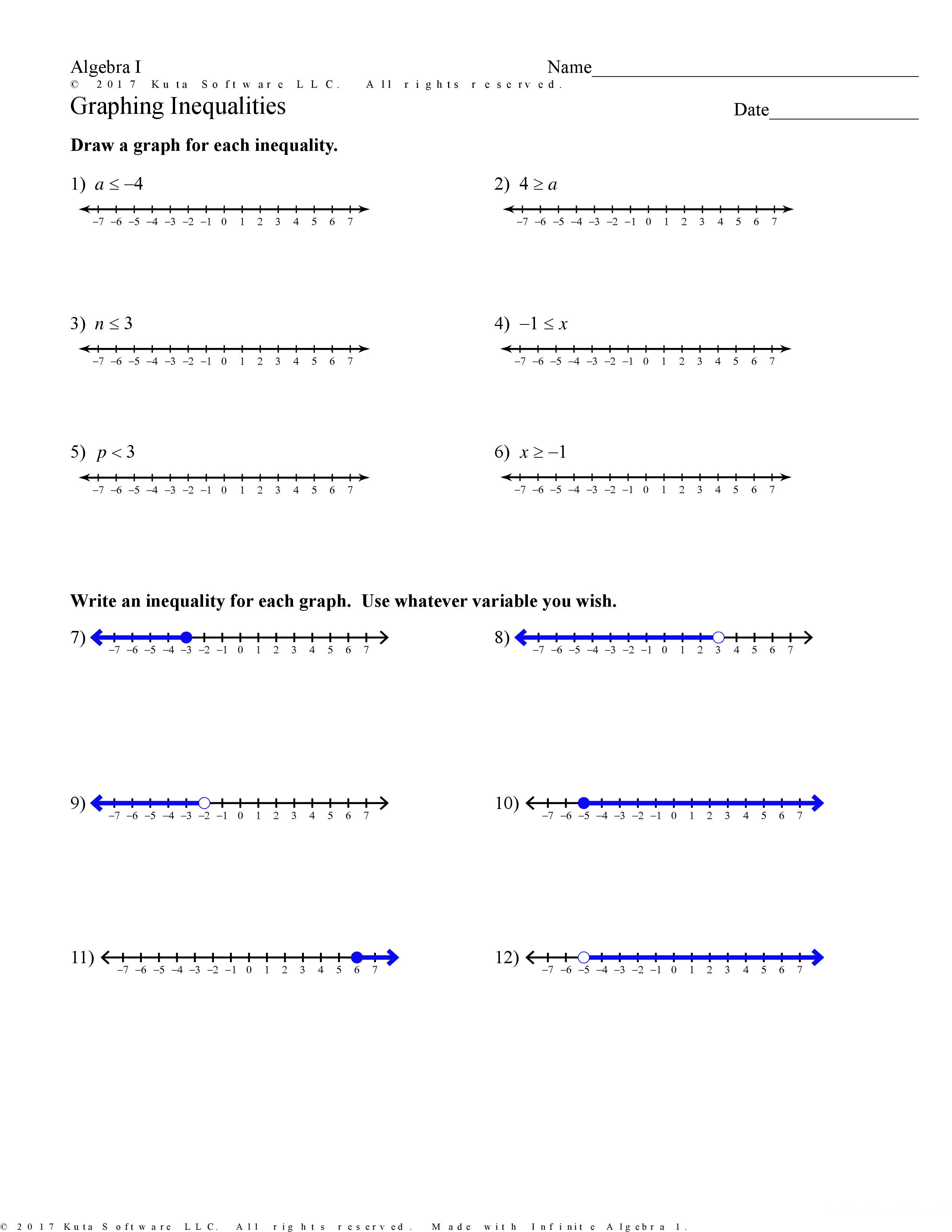Lesson 1 Practice: Graphing Inequalities
starstarstarstarstarstarstarstarstarstar
by Nicholas Nenni
| 12 Questions
Note from the author:
Block graphing inequalities1
2
3
4
5
6
7
8
9
10
11
12
1
1 pt
2
1 pt
3
1 pt
4
1 pt
5
1 pt
6
1 pt
7
1 pt
Write an inequality (use <= for less than or equal to, use >= for greater than or equal to)
8
1 pt
Write an inequality (use <= for less than or equal to, use >= for greater than or equal to)

9
1 pt
Write an inequality (use <= for less than or equal to, use >= for greater than or equal to)
10
1 pt
Write an inequality (use <= for less than or equal to, use >= for greater than or equal to)
11
1 pt
Write an inequality (use <= for less than or equal to, use >= for greater than or equal to)
12
1 pt
Write an inequality (use <= for less than or equal to, use >= for greater than or equal to)